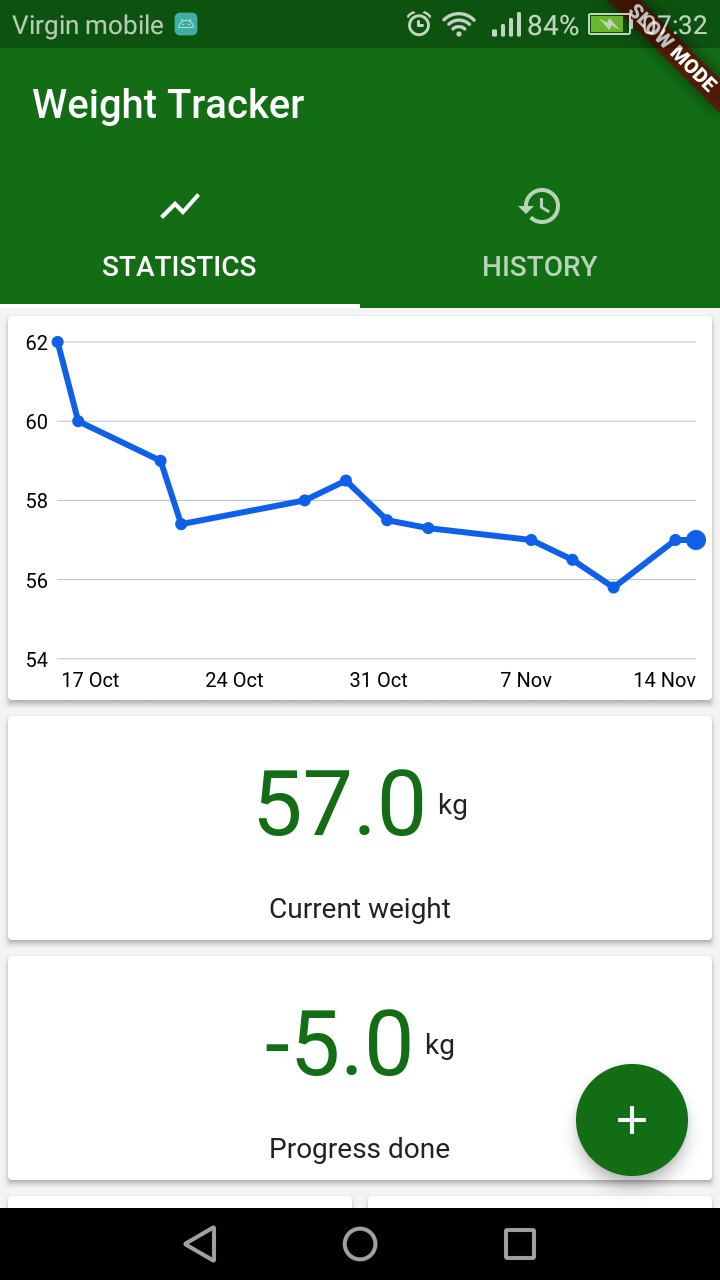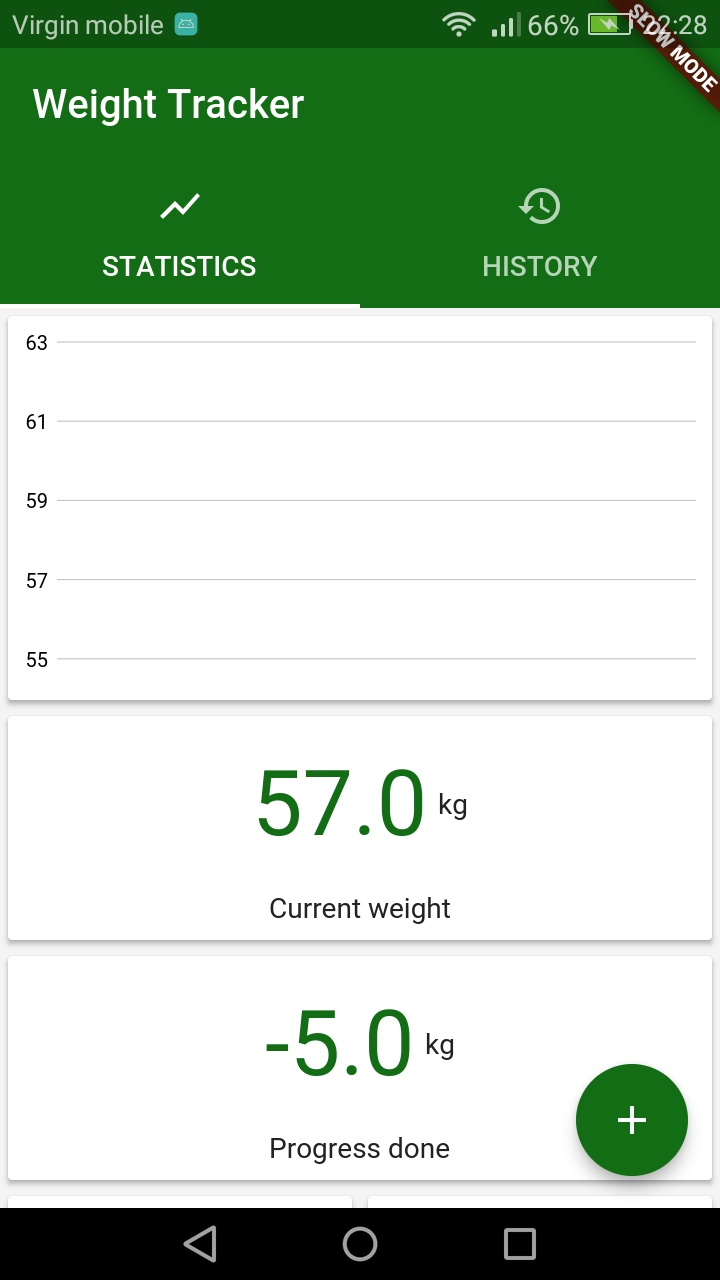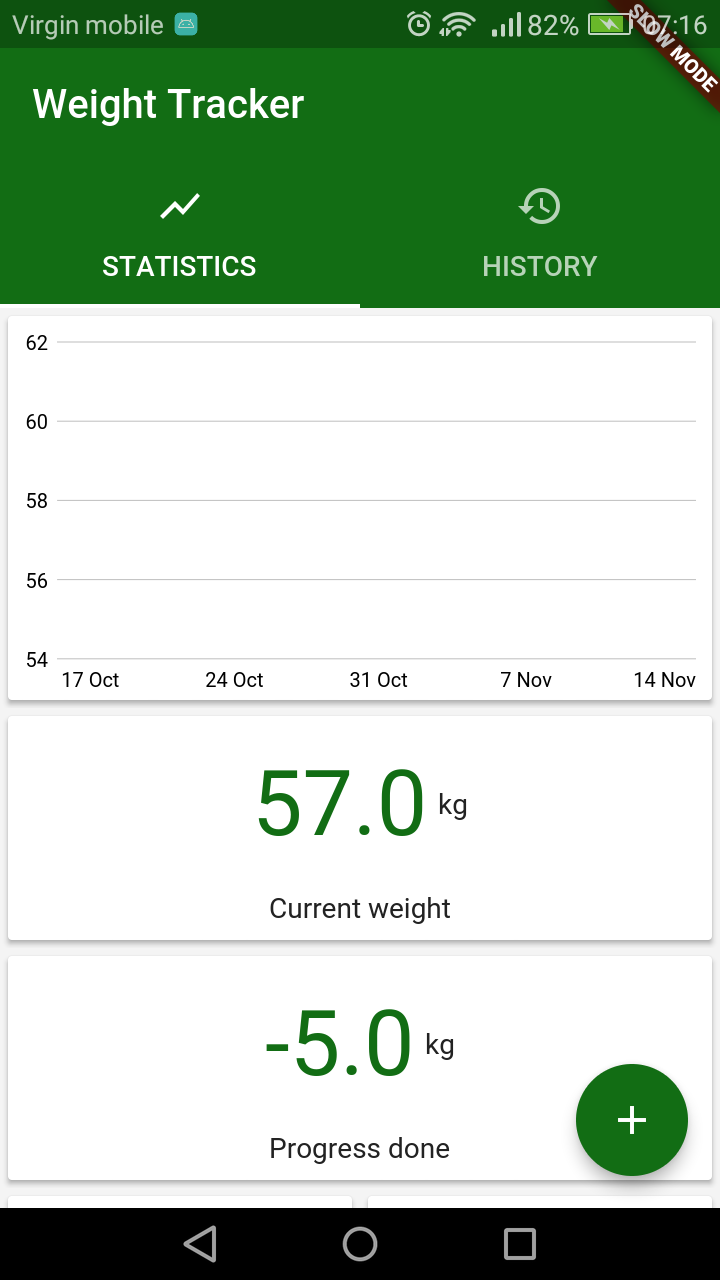Select Page

# Line Chart in Flutter – WeightTracker 6

In this post I will go through the process of creating line chart for my WeightTracker app. Since there is no official support for drawing charts yet, we will do it on our own. Expected result is a Widget displaying history of weight entries from last month. X axis will represent time (days) while Y axis will represent values (weights). Let’s get to it!## Introduction

At first, I created StatelessWidget which contains CustomPaint. This Widget can be used anywhere, in my case, I will display it inside a Card. I won’t discuss logic connected to preparing data since it is strictly connected to my business logic (see the full code if interested).

Next step is to create ChartPainter – a class that inherits from CustomPainter and is our most important class since there all painting will take place:

I created 4 fields (leftOffsetStart, topOffsetEnd, drawingWidth, drawingHeight) to calculate the area of drawings. We don’t want to use the full size we get because we need space for labels and small margins.
ShouldRepaint method ideally would check if we really should repaint our canvas but this is not important in the scope of this post.

. . .

## Calculating Y range

Since we are not using any charting library, we have to calculate the range of values we would like to present. I assumed, that my chart will have 5 horizontal lines each representing integer value.

Since there is no Pair in Dart and I wanted to return min and max value with one method, I had to use Tuple2 from Tuple package. To do that I needed to include Tuple package in pubspec.yaml file:

When it comes to calculating border values, at first I get ceil from max weight as max value. After that I calculate difference between min and max value. Then I need to change minimal value so that difference between min and max values is multiple of 4 (number of horizontal values – 1). Let’s crack that by example:
Let’s say that minimal weight in our data equals 7.4 and maximal 13.8.
maxLineValue = (13.8).ceil() = 14
difference = 14 – (7.4).floor() = 14 – 7 = 7
toSubtract = 4 – (difference % 4) = 4 – (7%4) = 4 – 3 = 1
minLineValue = (7.4).floor() – toSubtract = 7 – 1 = 6
Now having 5 lines, their labels would be accordingly: 6, 8, 10, 12, 14.

. . .

## Drawing horizontal lines and labels

Let’s get to drawing horizontal lines and labels. We’ll create grey Paint for lines and inside a for loop we will draw them on canvas:

At first, we need to calculate the weight step between every horizontal line. According to previous example it would be equal to 2:

Then we calculate the pixel difference between lines based on drawing height and number of lines. We will use that value so that every line will have Y-offset equal to numberOfLine*offsetStep :

When we get those, we can paint a line. To do that we need to call Canvas’ drawLine method with Offsets of starting and ending points. The ‘+5’ is there because I wanted to move chart a bit down:

The last thing we need to do is add labels to horizontal lines. Most of code are just aesthetic patches:

Right now this is what we got:. . .

## Drawing bottom labels

Process of creating bottom labels is pretty similar to left-side labels.
Instead of iterating through the number of lines, we do it by weeks (7 days), starting from today and moving 7 days backward with each iteration. To calculate left offset we simply divide drawing width by the number of days in total and multiplying it by number of days in current iteration:. . .

## Drawing actual data lines

At first we are creating blue Paint for our lines. Then for every entry we are going to draw line connecting that entry to the next one and we will draw circle (a dot) representing next entry. At last we will add bigger dot to the first entry, this dot will represent current state (since in my example first value is the most recent one and last value is the oldest):

To draw those lines, we need offsets representing values in our data. The first thing to do is getting relative x and y positions which tell us in what ‘part’ of drawing area point will be placed (from 0 to 1 where 0 is the beginning and 1 is the end). To calculate actual offset we need to get a starting position (our margin) and multiply the relative value with actual drawing size. As a result, we get Offset representing one entry:

. . .

## Wrapping up

Having everything together, we end up with chart that looks like this:And that’s it!Even if the full process may look complicated, once you get into it, it gets pretty easy, so until we have an official charting library we can still do charts on our own.I didn’t mention every aspect of my implementation (mostly connected to data transformation). If you are interested in more details, see my GitHub repository for more.Cheers! 🙂#### Marcin Szałek

Founder of Fidev

Flutter enthusiast since Alpha release in 2017. Believes that sharing is caring, which lead him to start a technical blog dedicated fo Flutter in its early days. Loves to see beautiful designs become real apps and is willing to help make it happen. Enjoys sunny beaches far from home.